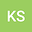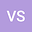On Solving Multi-Objective Fractional Linear Programming Problem with Intuitionistic Fuzzy Coefficients
••• Kirti Sharma,
• Vishnu Singh
Kirti Sharma
Visvesvaraya National Institute of Technology

Corresponding Author:[email protected]

Author ProfileVishnu Singh
Visvesvaraya National Institute of Technology Department of Basic Science
Author Profile## Abstract

In this paper, an approach for solving the Intuitionistic Fuzzy Multi-Objective Linear Fractional Programming Problem (IFMOLFPP) has been presented. The coefficients of objective functions, resources, and all the technological coefficients are taken to be a triangular intuitionistic fuzzy number (TIFN). In this paper, a component-wise optimization method has been adopted to handle the impreciseness. First, each intuitionistic fuzzy fractional objective is transformed into an equivalent crisp multiple fractional objectives, and then using the fuzzy programming approach, an optimal solution is obtained for every intuitionistic fuzzy fractional objective. Then, the optimal solution of each individual intuitionistic fuzzy fractional objective is used to transform it into a corresponding intuitionistic fuzzy linear objective and thus the original problem now transforms to Multi-Objective Intuitionistic fuzzy LPP which is further transformed into an Intuitionistic Fuzzy LPP with a single objective and the problem is then solved by using component-wise optimization and fuzzy goal programming approach. A numerical example is also presented to explain the methodology of the approach defined in this paper.